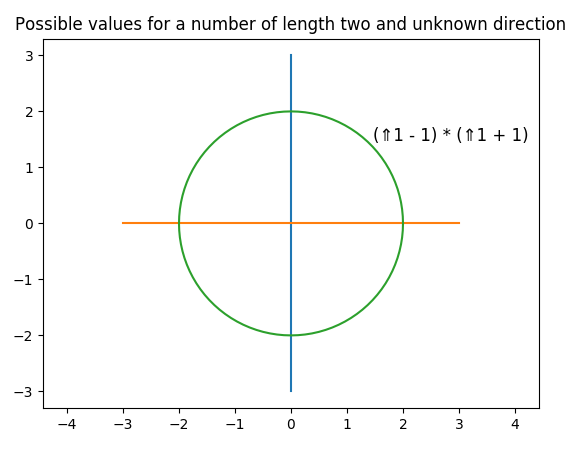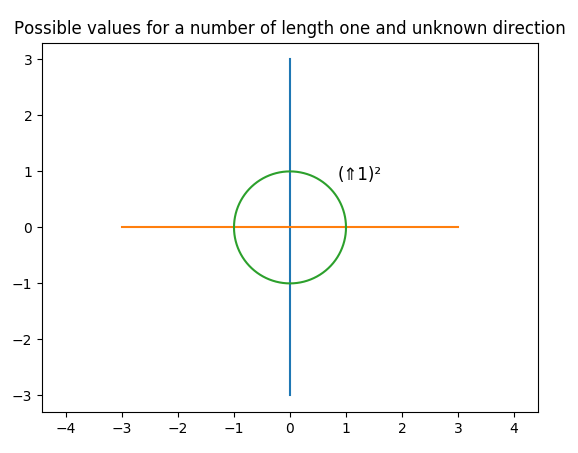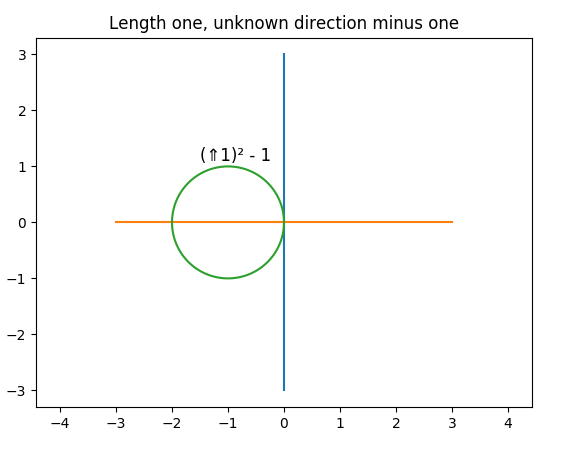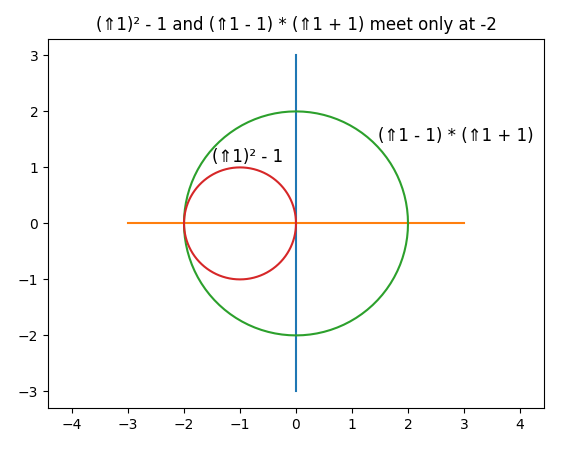## Why does complex multiplication have to be the way it is?

 In elementary school we learn about negative numbers, and how multiplication involving negative numbers works. There comes a moment that is unsatisfying for some kids: in class you may have been told the rules for what to do about the signs, but with no reason or justification. If you were lucky, you had a teacher who demonstrated why sign rules HAVE to be true. Something like this:

```      6 + -6 = 0
⇒ 2 * (6 + -6) = 2 * 0
⇒ (2 * 6) + (2 * -6) = 0
⇒ 2 * -6 = -12.
```

 We can do something like that for complex numbers. Instead of being handed the "sign" rule for complex multiplication "out of the blue", we can show why it has to be what it is. The idea will be to start with a few intuitive ideas about what complex addition might reasonably look like. From there, we will be able to derive something far less intuitive, namely what multiplication on complex numbers must look like.

 Say we think about "+" and "-" as specifying the "direction" of a number.

 We can easily imagine associating "+" with east; the positive numbers move to the right on a horizontal line.

 Similarly, we can associate "-" with west; the negative numbers move to the left on a horizontal line.

 We will do a slight switch in notation, and use little arrows to indicate signed numbers:

 ⇐47: negative 47

 ⇒83: positive 83.

 We will now take a leap, and allow other directions besides just east and west.

 This is the way we speak when we are giving directions: "To get to the nearest Starbucks, go three blocks east and then two blocks north".

 We can use arrows as souped-up number signs: For example, "⇑10" is "North 10", "⇓5" is "South 5", etc.

 We can use the "sign" of this new kind of number to represent any direction: "⇗20", "⇘30", "⇙40", "⇖50", etc.

 "The nearest big city is Syracuse, which is about ⇑60 miles from here."

 Let's call our numbers with souped-up signs "Compass numbers". We might then say that x and y are compass numbers like so:

```    x, y ∈ ℂ.
```

 (Having motivated the notation, we will at times make formulas below more visually natural by reverting to standard arithmetic notation for positive and negative numbers.)

 Just as with signed numbers at the beginning of this note, we have compass numbers A and B, and we want to know what the "sign" of A * B should be.

 Let's make a (hopefully) intuitive assumption that we know what it means to add A + B: "To get to the nearest Starbucks, go 3 blocks east and then 2 blocks north.." We view A and B as vectors on a plane, and add the vectors.

 To implement addition, it is convenient to pick two perpendicular directions such as ⇒1 and ⇑1, and break each number into a sum of those two compass numbers. For example,

```    ⇗2 = (⇑√ 2  ) + (⇒ √ 2  )
```

 Then we can add each of the directions separately, and convert the result back into a single compass number.

 Also, say we at least know how to multiply compass numbers by positive numbers. (Stretch or shrink in the number's direction.) For positive integers, this is consistent with addition: "A * 3" is equivalent to "A + A + A".

 And, as with signed numbers, we take as given that the length of a product is the product of the lengths.

 At this point we have gone as far as intuition can take us, and we are confronted with a dilemma: What if we have two compass numbers A and B that we want to multiply, and neither one of them is a positive number?

 We will start with a particular choice of A and B, for which we will be able to derive the direction that A*B must have.

 Surprisingly, it will turn out that this one key result will enable us to figure out what the direction A*B must be for any A and B in ℂ.

 Consider an interesting number "⇑1". This number is 1 with a heading of North.

 What should the "sign" of (⇑1) * (⇑1) be??

 Just as in the case of multiplying positive and negative numbers, there is a cute little derivation that tells you what the sign HAS to be!

 (We don't have to assume by fiat that (⇑1)2 = -1.)

 We know (x + 1) (x - 1) = x2 - 1. (Just multiply the left side out.)

 (N.B.: This requires an additional assumption. Our addition operation and our as-yet incompletely defined multiplication operation must follow the normal rules of arithmetic. I.e., they must constitute a field over the compass numbers: Commutativity, associativity, distributivity, etc.)

 Letting x = ⇑1, we have:

```    (⇑1 + 1) * (⇑1 - 1) = (⇑1)2 - 1
```

 Using compass-number addition, we can figure out where (⇑1 - 1) is (a vector pointing northwest), and where (⇑1 + 1) is (a vector pointing northeast).

 Each of these two vectors has length sqrt(2). So, the product on the left has length 2, but with an as-yet unknown "sign".

 This means that the left-hand side is somewhere on our compass number plane, on a circle of radius 2 centered at the origin:Similarly, for the right-hand side, (⇑1)2 has length 1 and is therefore somewhere on a circle of radius 1 centered at the origin. (Again, as yet we don't know what the "sign" is.)(⇑1)2 - 1 slides that whole circle to the left by 1:Well, the left-hand side and the right-hand side are equal, and so that means the two circles have to share at least one point, possibly more. Fortunately, the two circles meet at exactly one point! That one point is -2!And so, we know that (⇑1)2 - 1 = -2, or (⇑1)2 = -1.

 With that one fact in hand, we are in a position to deduce what multiplication of any two compass numbers must be.

 Say we are multiplying A and B. We represent each of them as sums of perpendicular directions, as in addition above:

```    A = (⇒a0) + (⇑a1)
B = (⇒b0) + (⇑b1)
```

 Then, we multiply these two sums term-by-term, to get four individual multiplications:

```    ((⇒a0) + (⇑a1)) * ((⇒b0) + (⇑b1)) =   ((⇒a0) * (⇒b0))
+ ((⇒a0) * (⇑b1))
+ ((⇑a1) * (⇒b0))
+ ((⇑a1) * (⇑b1))
```

 Three of the four terms involve multiplying by a positive or negative number, which we know how to do:

```    ((⇒a0) * (⇒b0)) = ⇒(a0 * b0)
((⇒a0) * (⇑b1)) = ⇑(a0 * b1)
((⇑a1) * (⇒b0)) = ⇑(a1 * b0)
```

 The fourth term is the product of a scaled version of ⇑1 by another scaled version of ⇑1.

 By the above result, we also know how to do that last multiplication:

```    ((⇑a1) * (⇑b1)) = ⇐(a1 * b1)
```

 Combining like terms, we end up with the following equation:

```    A * B = ⇒(a0*b0 - a1*b1) + ⇑(a0*b1 + a1*b0)
```

 If it so happens that B has length 1 and angle θ (the angle from the ray of positive numbers to B), we can express B in terms of sines and cosines:

```    B = ⇒cos(θ) + ⇑sin(θ)
```

 It turns out that the product A * B in that case is identical to the formula for rotating A by angle θ! So, it emerges that multiplying two complex numbers entails multiplying their lengths and adding their angles.

 That is the whole story. We have started with some intuitive initial assumptions about what it might mean to add two compass numbers, and from those we were able to derive the only possible definition of multiplication.